# Orifice Plate - Find Orifice Size

## How to calculate orifice diameter?

This calculator let you calculate the orifice plate diameter based on ISO 5167-2:2003 standard. This standard refers to the flow measurement with area reduction instruments, for circular pipes with the section completely filled with fluid.How to calculate orifice plate size?

The flow measurement using an orifice plate is based on the application of energy conservation to a flow, measuring the difference in pressure between two points (P1 and P2), at this points the flow has different speeds. This speed change is caused by a reduction in area. The orifice plate installed in the pipeline causes an increase in flow velocity and a corresponding decrease in pressure.

The equation that governs the use of these devices will be Bernoulli's equation in case of incompressible flows (liquids) or the first law of thermodynamics in compressible flows (gases). It should be noted however that the energy equation can be written in a very similar way to the Bernoulli equation under certain flow conditions, therefore the equation used in common practice comes from the Bernoulli equation and a factor to correct the compressibility of the fluid.

Orifice plates are still the most widely used type of flowmeter in the world today.

If you are interested in other orifice calculation like how to calculate flow through an orifice? please have a look in our other kind of orifice plate calculations :

Tagname
Plant
Area

## Fluid Data

Fluid
State
Dynamic Viscosity [cP] (mu)
Density [Kg/m3] (rho)
Temperature [ºC] (T)
Pressure In [bar a] (P1)
Ratio of Sp.Heats (kappa)
Molecular Weight (MW)
Compressiblity Factor (Z)
% of Water in Wet Steam (W)
Mass Flow [Kg/s] (qm)

## Instrument Data

Pressure Tappings
Pipe Diameter [mm] (Dm)
Bleed hole size [mm] (y)
Transmitter range [bar] (DP)
Linear Exp. Coef. Plate [1/ºC] (alphaplate)
Linear Exp. Coef. Pipe [1/ºC] (alphapipe)
Ambient Temperature [ºC] (T0)
Pipe Orientation

## Calculations

Int Pipe Diameter [mm] (D)
Pressure Ratio (P2/P1)
Pressure Drop Ratio (DP/P1)
Reynolds (ReD)
Reynolds Flow Regime
Steam Correction Factor (Fs)
Estimated Beta (Beta0)
Expansibility Factor (eps)
Discharge Coefficient (C)
Beta Ratio (Beta)
Beta % Error (errB)

Orifice Diameter at T [mm] (d)
Orifice Diameter at T0 [mm] (dm)

### Limits of Use

##### Pressure Ratio is correct.

We don't like spam either, but if we want to keep you informed we need you to provide us a minimal contact information. Thanks for your collaboration.

## Information and Definitions

Tagname

Tagname of the instrument. This is the identifier of the field device, which is normally given to the location and function of the instrument.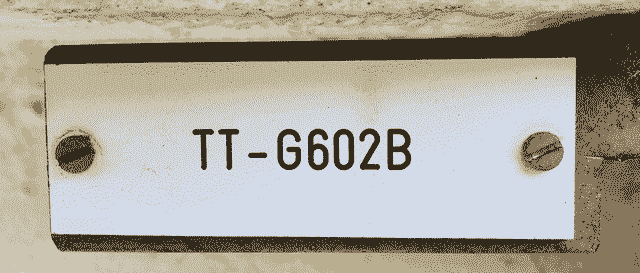Plant, Area and Notes

Information Referred to the physical installation of the instrument. Plant and Process Area where the instrument is installed. Notes about the instrument.

Fluid

Fluid Name or Composition. Fluid is called a type of continuous medium formed by some substance whose molecules have only a weak force of attraction.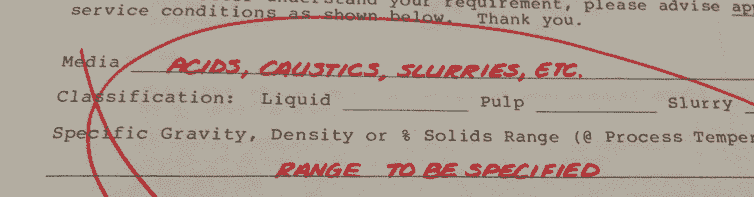A fluid is a set of particles that are held together by weak cohesive forces and the walls of a container; The term encompasses liquids and gases.

State

State of the matter. It could be Liquid, Gas or Steam.

Flow (qm)

Mass of a substance which passes per unit of time. Mass flow in Kg/s units, flowing through the pipe.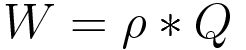...where W is Mass Flow Rate, rho is Density and Q is Volumetric Flow Rate.

Temperature (T)

Operating Temperature of the fluid in Celsius units. The flowing temperature is normally measured downstream from the orifice and must represent the average temperature of the flowing stream in degrees Celsius. Temperature has two effects on volume. A higher temperature means a less dense gas and higher flows, but when this higher flow is corrected to base temperature, the base flow is less.

Pressure In (P1)

Considering the direction of the fluid, we define P1 as the pressure (gauge or absolut) existing in the pipeline before the restriction orifice.Pressure has two effects on volume. The higher pressure makes the gas denser so less volume flows through the meter. However, when the volume is expanded to base pressure, the volume is increased.

Dynamic Viscosity (mu)

Viscosity is the measure of a fluid's resistance to flow. Dynamic viscosity is a measure of internal resistance.It measures the tangential force per unit area required to move one horizontal plane with respect to an other plane.It is commonly expressed, particularly in ASTM standards, as centipoise (cP) since the latter is equal to the SI multiple millipascal seconds (mPa·s).The viscosity of a fluid is highly temperature dependent.

Density (rho)

Density is the relation of mass and volume.The density of a material varies with temperature and pressure. This variation is typically small for solids and liquids but much greater for gases.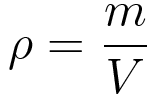...where rho is density, m is mass and V is volume.

Ratio of Sp.Heats (kappa)

Ratio of the heat capacity at constant pressure (CP) to heat capacity at constant volume (CV). It is sometimes also known as the isentropic expansion factor and is denoted by γ (gamma) for an ideal gas or κ (kappa), the isentropic exponent for a real gas. .% of Water in Wet Steam (W)

Wet steam is a mixture of steam and liquid water. It exists at a saturation temperature containing more than 5% water. It is said to be a two-phase mix: steam contains droplets of water that have not changed phase. % in volume of water present in steam.

Pressure Tappings

The pressure taps are placed before of the orifice plate (upstream) represented with P1 and after the orifice plate (downstream) represented with P2. In ISO 5167 there are three choices for the tappings: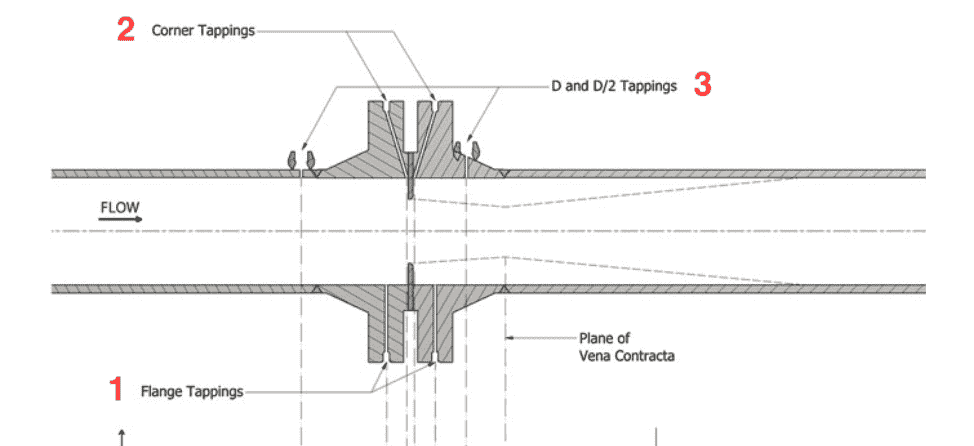1. Flange tappings

They are used more frequently because it is one of the simplest configurations and it is not necessary to drill the pipe. The high pressure outlet (H) is located 1 inch (25.4 mm) before the plate and the low pressure (L) 1 inch (25.4 mm) after the plate. With a tolerance of 0.5 mm when Beta larger than 0.6 and D less than 150 mm and 1 mm in other cases. They cannot be used for pipe size of less than 35 mm diameter. Since the venacontracta may be closer than 25 mm from the orifice plate.

2. Corner tappings

In this case the pressure taps are made directly at the edge of the plate by piercing the flange through independent holes made with a small inclination. These taps are similar in many respects to flange taps, except that the pressure is measured at the ‘Corner’ between the orifice plate and the pipe wall. Can be used for diameters of less than 50 mm. The diameter of the holes a must be between 0.005 D and 0.03 D for Beta less than 0.65, and between 0.1 D and 0.02 D for Beta greater than 0.65.

3. Radius tappings (D and D/2)

The taps are made in the pipeline at fixed distances of 1 D before the orifice plate and 1⁄2 D after the orifice plate. There is however a tolerance of 0.9 D to 1.1 D for upstream tap and for the downstream tap we will use 0.48 D to 0.52 D for Beta Ratio less than 0.6, and 0.49 D to 0.51 D, for Beta Ratio larger than 0.6.

As a rule of thumb Corner tappings are recommended for sizes up to 1 1⁄2”, Flange tappings from 2" to 16" and Radius tappings for higher sizes.

Orifice plate edge shape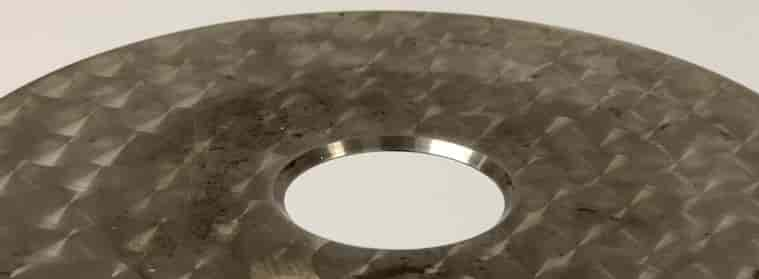The edge of the orifice plate generally has a special shape in order to minimize contact between the fluid and the orifice plate. This is usually done by chamfering at an angle of approximately 45 degrees at the edge of the orifice so that the edge is as narrow as possible, keeping the resistance of the plate.

Pipe Diameter (Dm)

Inside diameter of the pipe. All process calculations are based on the volume of the pipe which is the function of internal diameter of the pipe. As per standards, any pipe is specified by two non-dimensional numbers Nominal Diameter (in Inches as per American Standards or mm as per European standards) and Schedule (40, 80, 160,...). The outer diameter of the pipe is the diameter of outer surface of the pipe.

Bleed hole size (y)

Size of the purge orifice in mm. The bleed hole is required in gas flow services where we can have trapped liquid. Bleed hole is not recommended in dirty fluid service or slurries as the hole could be plugged.

Transmitter range (DP)

We can design our orifice plate based on Pressure transmitter range, in this case we will introduce the span of the transmitter in the cell.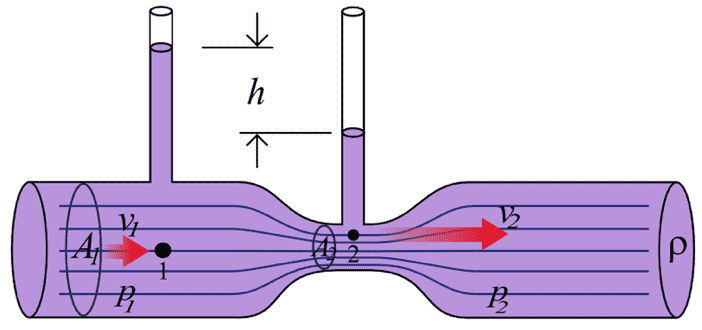We can design our orifice plate based on Pressure transmitter range, in this case we will introduce the span of the transmitter in the cell.

Linear Expansion Coefficient for Plate (alphaplate) and for Pipe (alphapipe)

The coefficient of Linear thermal expansion describes how the length of an object changes with a change in temperature. Specifically, it measures the fractional change in size per degree change in temperature at a constant pressure.

Ambient Temperature (T0)

Ambient temperature is the air temperature of any object or environment where equipment is stored. The adjective ambient means "relating to the immediate surroundings." Also sometimes referred to as the ordinary temperature or the baseline temperature, this value is important for system design and thermal analysis.

Pipe Orientation

In this cell you can tell us how the orifice plate regarding pipe is installed it can be installed horizontal or vertical.

HorizontalVertical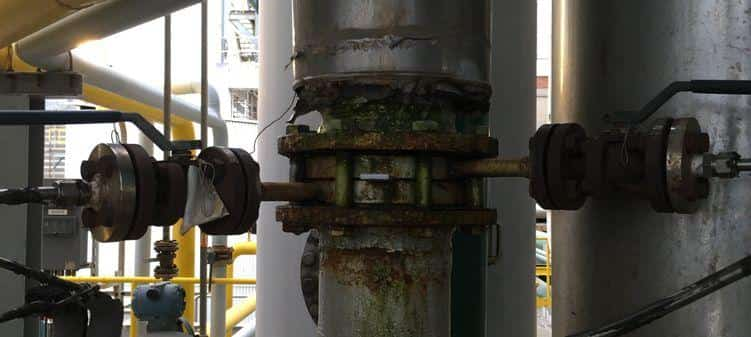Int Pipe Diameter (D)

Internal pipe diameter in mm. It has in consideration the thermal expansion of the pipe.

Pressure Ratio (PR)

Pressure Ratio at which the discarge coefficient determined has the value C.

Pressure Drop Ratio (PDR)

Pressure Drop Ratio (PDR=DP/P1) let you know the need to use compressible equations. For PDR less than 0.2 the change in gas density is small, and the assumption of imcompressible flow can me made. For PDR greater than 0.4, the density change is large and compressible flow should be assumed.

Reynolds (ReD) and Reynolds Flow Regime

The Reynolds number (Re) is an important dimensionless quantity in fluid mechanics used to help predict flow patterns in different fluid flow situations. It is defined as :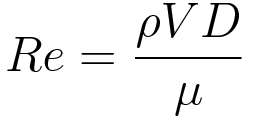...where rho is density, V is velocity of fluid in pipe, D is pipe diameter and mu is dynamic viscosity.

At low Reynolds numbers, flows tend to be dominated by laminar (sheet-like) flow, while at high Reynolds numbers turbulence results from differences in the fluid's speed and direction, which may sometimes intersect or even move counter to the overall direction of the flow.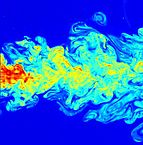Image by C. Fukushima and J. Westerweel, Technical University of Delft, The Netherlands

There are in general three types of fluid flow in pipes

Laminar : 0 <= Reynolds Number <= 2300

Transitional : 2100 <= Reynolds Number <= 4000

Turbulent : 4000 <= Reynolds Number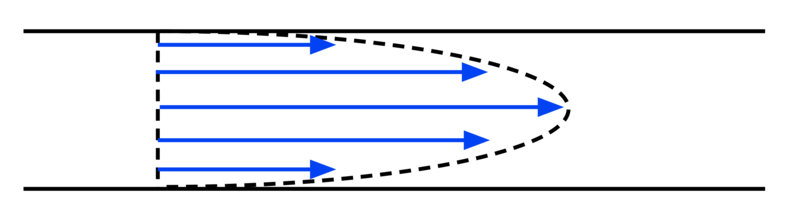Steam Correction Factor (Fs)

Only applies to Steam service. It only applies to the Steam service. Factor used in the calculation of the Beta Ratio to take into account the amount of water in the steam flow.

Expansibility Factor (eps)

Also called the expansion factor. The expansion factor corrects for the density change between the measured tap density and the density at the plane of the orifice face.

Discharge Coefficient (C)

The discharge coefficient is a dimensionless number used to characterise the flow and pressure loss behaviour of nozzles and orifices in fluid systems.It depends on the orifice shape. The discharge coefficient can be obtained for any differential-pressure meter and any installation by calibrating it in a flowing fluid: for a particular orifice meter the discharge coefficient is a function of the Reynolds number.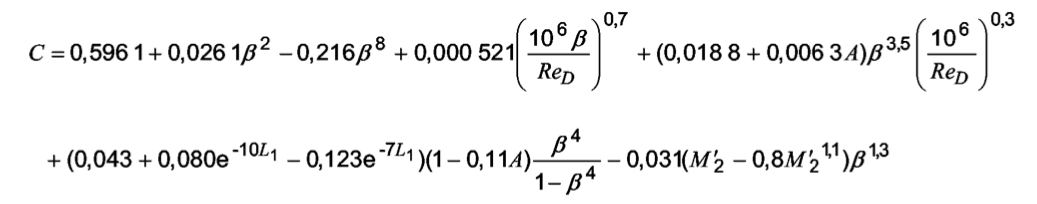Over many years of experiment it has been found that the discharge coefficient can be predicted within a defined uncertainty provided that the orifice meter (i.e. the orifice plate and pipework) are constructed within the standards. If the discharge coefficient is to be used for an orifice meter without calibrating it in a flowing fluid, the discharge coefficient is usually taken from a published discharge coefficient equation. Therefore, the discharge coefficient equation is very important for orifice plates: an error of 0.1 % in discharge coefficient gives an error of 0.1 % in many flow measurements of natural gas. ISO 5167-1:2003 provides an equation for the orifice discharge coefficient calculation, Cd, as a function of Beta Ratio, Reynolds number, L1 and L2, where L1 is the distance of the upstream pressure tap from the orifice plate and L2 is the distance of the downstream pressure tap from the orifice plate.

Beta Ratio (Beta) and Estimated Beta (Beta0)

Beta Ratio is the ratio between the line inner diameter to bore size of the orifice. The flow coefficient is found to be stable between beta ratio of 0.2 to 0.7 below which the uncertainity in flow measurement increases. An orifice plate beta ratio of 0.6 means that the orifice plate bore diameter is 60% of the pipe internal diameter.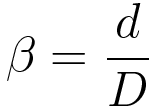...where Beta is Beta Ratio, d is orifice diameter and D is orifice diameter.

Beta % Error (errB)

Error obtained in the calculation of the final Beta Ratio (Beta) with respect to the Estimated Beta (Beta0).

Orifice Diameter at T (d)

Inside diameter of the orifice plate at fluid's temperature.

Orifice Diameter at T0 (dm)

Inside diameter of the orifice plate at ambient temperature.

Limits of Use

Calculation's Limits of Use according to ISO 5167-2:2003. Standard orifice plates shall only be used in accordance with this conditions. See page point 5.3.1 of ISO 5167-2:2003.

## Orifice Plate Flow Meter References

1. International Organization of Standards (ISO 5167-1). 2003. Measurement of fluid flow by means of pressure differential devices inserted in circular cross-section conduits running full — Part 1: General principles and requirements.

2. International Organization of Standards (ISO 5167-2) 2003. Measurement of fluid flow by means of pressure differential devices inserted in circular cross-section conduits running full -- Part 2: Orifice plates.

3. American Society of Mechanical Engineers (ASME). 2001. Measurement of fluid flow using small bore precision orifice meters. ASME MFC-14M-2001.

4. U.S. Dept. of the Interior, Bureau of Reclamation, 2001 revised, 1997 third edition, Water Measurement Manual.

5. Michael Reader-Harris (2015) Orifice Plates and Venturi Tubes.

6. Miller, R. W., Flow Measurement Handbook, 3rd ed., McGraw-Hill, New York, 1996.

7. American Gas Association, AGA Gas Measurement Manual, American Gas Association, New York.

8. Wikipedia

9. Corrosionpedia

10. Orifice Plates and Venturi Tubes (2015) - Michael Reader-Harris

11. EMERSON Fundamentals of Orifice Meter Measurement

12. Search Data Center

x